首页 > 酒店 > 南京市> 南京希尔顿酒店

### 南京希尔顿酒店875

1.0分 /（112人评价）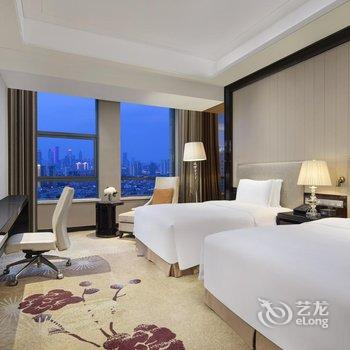•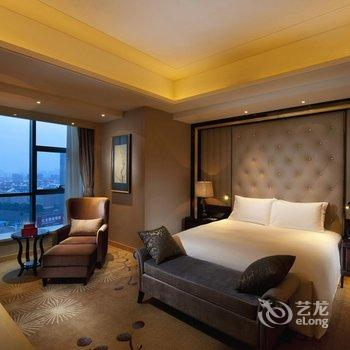•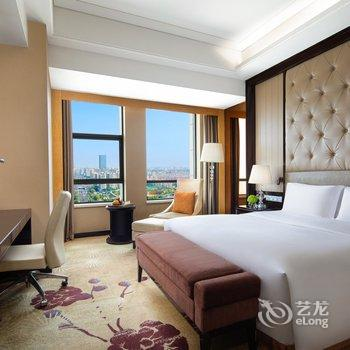•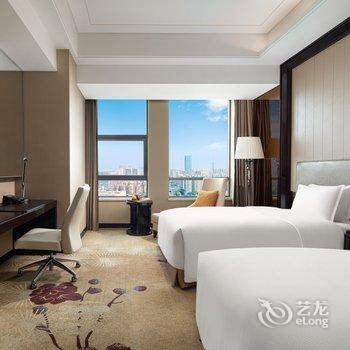•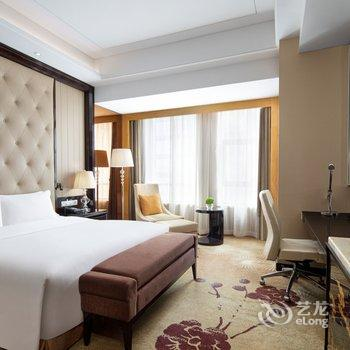•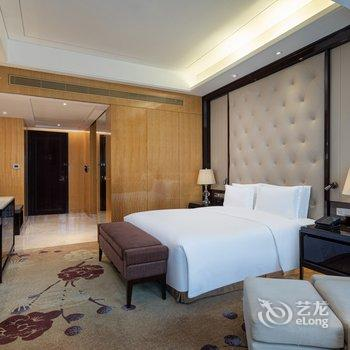•#### 希尔顿双床房

• 不含早（内宾）
• 双床1.1米
• 有窗
• 免费无线
• ￥875
• 有房
• 预付
• 预订
• 标准价
• 双床1.1米
• 有窗
• 免费无线
• ￥886
• 有房
• 预付
• 预订
• 标准价
• 双床1.1米
• 有窗
• 免费无线
• ￥886
• 有房
• 担保
• 预订
• 含单早（内宾）
• 双床1.1米
• 有窗
• 免费无线
• ￥953
• 有房
• 预付
• 预订
• 标准价
• 双床1.1米
• 有窗
• 免费无线
• ￥965
• 有房
• 担保
• 预订
• 标准价
• 双床1.1米
• 有窗
• 免费无线
• ￥1,045
• 有房
• 担保
• 预订
• 标准价
• 双床1.1米
• 有窗
• 免费无线
• ￥1,045
• 有房
• 预付
• 预订
• 标准价
• 双床1.1米
• 有窗
• 免费无线
• ￥1,241
• 有房
• 担保
• 预订#### 希尔顿大床房

• 不含早（内宾）
• 大床1.8米
• 有窗
• 免费无线
• ￥875
• 有房
• 预付
• 预订
• 标准价
• 大床1.8米
• 有窗
• 免费无线
• ￥886
• 有房
• 预付
• 预订
• 标准价
• 大床1.8米
• 有窗
• 免费无线
• ￥886
• 有房
• 担保
• 预订
• 标准价
• 大床1.8米
• 有窗
• 免费无线
• ￥965
• 有房
• 预付
• 预订
• 标准价
• 大床1.8米
• 有窗
• 免费无线
• ￥965
• 有房
• 担保
• 预订
• 标准价
• 大床1.8米
• 有窗
• 免费无线
• ￥1,045
• 有房
• 担保
• 预订
• 标准价
• 大床1.8米
• 有窗
• 免费无线
• ￥1,045
• 有房
• 预付
• 预订
• 标准价
• 大床1.8米
• 有窗
• 免费无线
• ￥1,241
• 有房
• 担保
• 预订#### 希尔顿豪华双床房

• 不含早（内宾）
• 双床1.1米
• 有窗
• 免费无线
• ￥978
• 有房
• 预付
• 预订
• 标准价
• 双床1.1米
• 有窗
• 免费无线
• ￥991
• 有房
• 预付
• 预订
• 标准价
• 双床1.1米
• 有窗
• 免费无线
• ￥991
• 有房
• 担保
• 预订
• 标准价
• 双床1.1米
• 有窗
• 免费无线
• ￥1,070
• 有房
• 预付
• 预订
• 标准价
• 双床1.1米
• 有窗
• 免费无线
• ￥1,070
• 有房
• 担保
• 预订
• 标准价
• 双床1.1米
• 有窗
• 免费无线
• ￥1,150
• 有房
• 担保
• 预订
• 标准价
• 双床1.1米
• 有窗
• 免费无线
• ￥1,150
• 有房
• 预付
• 预订
• 标准价
• 双床1.1米
• 有窗
• 免费无线
• ￥1,346
• 有房
• 担保
• 预订#### 希尔顿豪华大床房

• 不含早（内宾）
• 大床1.8米
• 有窗
• 免费无线
• ￥978
• 有房
• 预付
• 预订
• 标准价
• 大床1.8米
• 有窗
• 免费无线
• ￥991
• 有房
• 预付
• 预订
• 标准价
• 大床1.8米
• 有窗
• 免费无线
• ￥991
• 有房
• 担保
• 预订
• 标准价
• 大床1.8米
• 有窗
• 免费无线
• ￥1,070
• 有房
• 预付
• 预订
• 标准价
• 大床1.8米
• 有窗
• 免费无线
• ￥1,070
• 有房
• 担保
• 预订
• 标准价
• 大床1.8米
• 有窗
• 免费无线
• ￥1,150
• 有房
• 担保
• 预订
• 标准价
• 大床1.8米
• 有窗
• 免费无线
• ￥1,150
• 有房
• 预付
• 预订
• 标准价
• 大床1.8米
• 有窗
• 免费无线
• ￥1,346
• 有房
• 担保
• 预订#### 希尔顿行政双床房

• 不含早（内宾）
• 双床1.1米
• 有窗
• 免费无线
• ￥1,151
• 有房
• 预付
• 预订
• 标准价
• 双床1.1米
• 有窗
• 免费无线
• ￥1,166
• 有房
• 担保
• 预订
• 标准价
• 双床1.1米
• 有窗
• 免费无线
• ￥1,166
• 有房
• 预付
• 预订
• 标准价
• 双床1.1米
• 有窗
• 免费无线
• ￥1,245
• 有房
• 预付
• 预订
• 标准价
• 双床1.1米
• 有窗
• 免费无线
• ￥1,245
• 有房
• 担保
• 预订
• 标准价
• 双床1.1米
• 有窗
• 免费无线
• ￥1,325
• 有房
• 担保
• 预订
• 标准价
• 双床1.1米
• 有窗
• 免费无线
• ￥1,325
• 有房
• 预付
• 预订#### 希尔顿行政大床房

• 不含早（内宾）
• 大床1.8米
• 有窗
• 免费无线
• ￥1,151
• 有房
• 预付
• 预订
• 标准价
• 大床1.8米
• 有窗
• 免费无线
• ￥1,166
• 有房
• 担保
• 预订
• 标准价
• 大床1.8米
• 有窗
• 免费无线
• ￥1,166
• 有房
• 预付
• 预订
• 标准价
• 大床1.8米
• 有窗
• 免费无线
• ￥1,245
• 有房
• 预付
• 预订
• 标准价
• 大床1.8米
• 有窗
• 免费无线
• ￥1,245
• 有房
• 担保
• 预订
• 标准价
• 大床1.8米
• 有窗
• 免费无线
• ￥1,325
• 有房
• 担保
• 预订
• 标准价
• 大床1.8米
• 有窗
• 免费无线
• ￥1,325
• 有房
• 预付
• 预订#### 希尔顿精致套房

• 标准价
• 大床1.8米
• 有窗
• 免费无线
• ￥1,341
• 有房
• 担保
• 预订
• 标准价
• 大床1.8米
• 有窗
• 免费无线
• ￥1,420
• 有房
• 担保
• 预订
• 标准价
• 大床1.8米
• 有窗
• 免费无线
• ￥1,499
• 有房
• 担保
• 预订
• 标准价
• 大床1.8米
• 有窗
• 免费无线
• ￥1,341
• 满房
• 预付
• 满房#### 希尔顿豪华套房

• 不含早（内宾）
• 大床1.8米
• 有窗
• 免费无线
• ￥2,244
• 有房
• 预付
• 预订
• 标准价
• 大床1.8米
• 有窗
• 免费无线
• ￥2,274
• 有房
• 担保
• 预订
• 标准价
• 大床1.8米
• 有窗
• 免费无线
• ￥2,274
• 有房
• 预付
• 预订
• 含单早（内宾）
• 大床1.8米
• 有窗
• 免费无线
• ￥2,322
• 有房
• 预付
• 预订
• 标准价
• 大床1.8米
• 有窗
• 免费无线
• ￥2,353
• 有房
• 担保
• 预订
• 标准价
• 大床1.8米
• 有窗
• 免费无线
• ￥2,432
• 有房
• 担保
• 预订#### 大使套房

• 标准价
• 大床2米
• 未知
• 免费无线
• ￥4,606
• 有房
• 担保
• 预订
• 标准价
• 大床2米
• 未知
• 免费无线
• ￥4,685
• 有房
• 担保
• 预订
• 标准价
• 大床2米
• 未知
• 免费无线
• ￥4,764
• 有房
• 担保
• 预订

• 上网服务
免费wifi
• 酒店电话
025-86658888
• 停车场
免费停车场
• 开业时间
酒店开业时间 2011-11-01 新近装修时间 2022-06-25
• 酒店设施